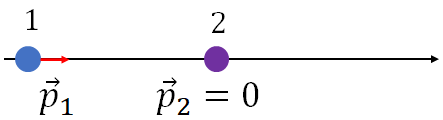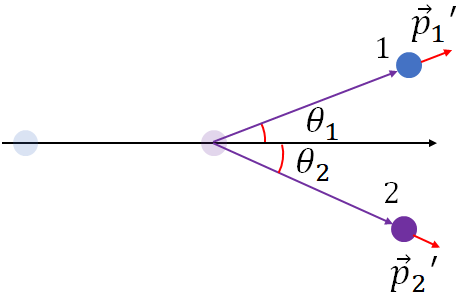﻿ Linear momentum

# Momentum

When an object is in motion, we say it has momentum. Momentum is a physical quantity that is powerful to study motion of objects involving collision. You will see that like energy, momentum is also a conserved quantity.

### Linear momentum or momentum

If an object of mass, $m$ moving with a velocity, $\vec v$, then the momentum of the object is defined as

$\vec p=m\vec v$

Momentum is a vector and its direction is same as the direction of the object's motion. If you just say momentum you actually refers to linear momentum as opposed to another type of it called angular momentum.

Unit of momentum is $kg.m/s$ or $N.s$.

#### Force and momentum

To change the momentum of an object, you need to change the velocity of the object. Changing velocity means, accelerating the object. To accelerate an object you need to apply a force on the object. So, to change the momentum of an object, a force needs to be applied on the object. We can derive an equation that relates force and momentum change, by using Newton's second law.

Assume an object is moving with some velocity, say the initial velocity, $\vec v_i$. Now you are applying a net force, $\vec F$ on the object for a time period, $\Delta t$. The force accelerates the object, and at the end of the time, let the object's velocity is $\vec v_f$. Writing Newton's second law,

$\vec F=m\vec a=m\dfrac{\Delta \vec v}{\Delta t}=m\dfrac{(\vec v_f-\vec v_i)}{\Delta t}=\dfrac{m\vec v_f-m\vec v_i}{\Delta t}=\dfrac{\vec p_f-\vec p_i}{\Delta t}$
(or)
$\vec F=\dfrac{\Delta \vec p}{\Delta t}$

i.e., the force applied on an object is just the change in momentum over time or the rate of change of momentum of the object. This equation is actually the original form of Newton's second law as formulated by Newton.

#### Isolated system

If you have two or more objects, we call that a system of objects. If in a system, where only forces acting on the objects are the forces exert on one another, then we call that system, an isolated system. So, in an isolated system, there is no external force acting on the objects other than the forces on one another. Since objects exert equal and opposite force on one another according to Newton's third law, if you add all the forces acting on the objects, you will get zero. i.e., the net force acting in an isolated system is zero.

#### Law of conservation of momentum

We can prove that total momentum of an isolated system is conserved. i.e., if objects of an isolated system is involved in a collision, the total momentum of the system before collision is equal to the total momentum after the collision. This is called the law of conservation of momentum. Total momentum of the system is the sum of the momenta of the objects of the system.

To derive the law of conservation of momentum, we consider a system of two objects collide with each other. Let $\vec v_1$, and $\vec v_2$ are the velocities of object 1 and object 2 and $\vec p_1$ and $\vec p_2$ are their momentum, before the collision:

$\vec p_1 = m_1 \vec v_1$ and $\vec p_2 = m_2 \vec v_2$

Total momentum of the system before collision is the sum of the momentum of object 1 and object 2:

$\vec p_i=\vec p_1+\vec p_2$.

Let $\vec v_1'$, and $\vec v_2'$ and are the velocities of the objects after the collision and $\vec p_1'$ and $\vec p_2'$, their momenta after the collision:

$\vec p_1'=m_1 \vec v_1'$ and $\vec p_2'=m_2 \vec v_2'$

Now, the total momentum of the system after the collision is,

$\vec p_f=\vec p_1'+\vec p_2'$.

During the collision, object 1 exerts a force, $\vec F_1$ on object 2, and object 2 exerts a force, $\vec F_2$ on object 1 with same magnitude but in the opposite direction (Newton's third law):

$\vec F_1=-\vec F_2$.

Now, we will use the force momentum relation. Force, $\vec F_{1}$ on object 2 changes its momentum and the force, $\vec F_{2}$ on object 1, changes its momentum.

$\vec F_{1}=\dfrac{\Delta \vec p_2}{\Delta t}$ and $\vec F_{2}=\dfrac{\Delta \vec p_1}{\Delta t}$

Substituting these in the above equation, we get,

$\dfrac{\Delta \vec p_2}{\Delta t}=-\dfrac{\Delta \vec p_1}{\Delta t}$

(or)

$\dfrac{\vec p_2'- \vec p_2}{\Delta t}=-\dfrac{\vec p_1'-\vec p_1}{\Delta t}$

Canceling, $\Delta t$ and rearranging, we have,

$\vec p_1+\vec p_2=\vec p_1'+\vec p_2'$.

i.e., $\vec p_i=\vec p_f$.

Total momentum of the system before the collision is equal to total momentum after the collision.

Note that, we used an isolated system to derive the law of conservation of momentum. In an isolated system, there is no external force acting on the objects of the system. The good thing is we can approximate all system as isolated system if we consider the kinematics of the objects immediately after the collision, and we can apply the momentum conservation law.

#### One dimensional collision

One dimensional collision means, the objects involves in a collision move on a line before and after the collision. If the collision is 1-D, then you don't have to use the vector notation, but just be careful about the sign of the velocity vectors as in 1-D motion, sign shows the direction of a vector. Therefore if two objects involve in a collision, then the momentum conservation becomes

$p_i=p_f$.

(or)

$m_1v_1+m_2v_2=m_1v_1'+m_2v_2'$.

#### Impulse

When two objects are in collision, there is a brief period of time, the objects exert force on one another. The force on an object multiplied with the impact time is called the impulse.

Impulse imparted to an object by a force $(\vec F)$ is

$Impulse=\vec F \Delta t$

By using the force-momentum relation, $\vec F=\dfrac{\Delta \vec p}{\Delta t}$, we can write,

$Impulse=\Delta \vec p$

i.e., impulse is just the change in momentum. So you can use either of the above formula to find the impulse.
Impulse is a vector and its direction is the direction of the force.
Applying a larger force for a short time produces same change momentum or impulse as applying a small force for a long time.

### Types of collisions

There are two types of collisions: elastic and inelastic collision. But in an inelastic collision, kinetic energy is not conserved.

#### Elastic collision:

Momentum is conserved in all types of collision. In an elastic collision, kinetic energy is also conserved. Therefore, for two objects involve in an 1-D elastic collision,

$m_1v_1+m_2v_2=m_1v_1'+m_2v_2'$.

and

$KE_1+KE_2=KE'_1+KE'_2$

$KE_1$ and $KE_2$ are the kinetic energies of the objects before the collision and $KE'_1$ and $KE'_2$ are the kinetic energies of the objects after the collision.

You can combine the above two equations and can get one equation for 1-D elastic collision:

$v_1-v_2=-(v'_1-v'_2)$

Collision between hard objects is generally elastic. That is, collision between billiard balls and between atoms or molecules. During an elastic collision, no heat or other energy is produced, so there is no loss in kinetic energy.

#### Inelastic collision:

In an inelastic collision, only the momentum is conserved, kinetic energy is not conserved. During such a collision, there is a loss or gain in kinetic energy of the system occurs that makes the kinetic energy not conserved. Further an inelastic collision can be, completely inelastic or partially inelastic. In a completely inelastic collision, the colliding objects stick together, but in a partially inelastic collision, the objects separate after the collision.

#### 2-D collision

In a two dimensional (2-D) collision, the colliding objects move away from their original line of path after the collision. Consider, there are two objects on the $x$ axis. Object 1 is moving in the $+x$ direction with a speed $v_1$ and object 2 is at rest.Before collision: object 1 is moving and object 2 is in rest. Let $\vec p_1$ is the momentum of object 1 and $\vec p_2$ is that of object 2. Therefore, we have

$p_1=m_1v_1$ and $p_2=0$; the magnitude of the momenta.After collision: object 1 is moving at an angle $\theta_1$ and object 2 at $\theta_2$. After the collision, object 1 move at an angle $\theta_1$ above the $x$ axis with speed $v_1'$ and object 2 at an angle $\theta_2$ below the $x$ axis with speed $v_2'$. If you take, $\vec p_1'$ and $\vec p_2'$ are their momenta after the collision, we have

$p_1'=m_1v_1'$ and $p_2'=m_1v_2'$; the magnitude of the momenta.

Since momentum is a vector, $x$ and $y$ components of momentum is independent of each other. Therefore, momentum is conserved along the $x$ axis and along the $y$ axis separately. So, we will have two momentum conservation equations, one for the $x$ direction and the other for the $y$ direction.

First let us find the $x$ and the $y$ components of the momenta.

Before the collision: Since the momentum of object 1 is along the $x$ axis:

$p_{1x}= m_1v_1$ and $p_{1y}= 0$

Since object 2 is at rest, both of its momentum components are zero:

$p_{2x}= 0$ and $p_{2y}= 0$

After the collision:

For object 1, from the figure, we can write

$p_{1x}'=m_1v_1'\cos\theta_1$ and $p_{1y}'=m_1v_1'\sin\theta_1$

And for the object 2,

$p_{2x}'=m_2v_2'\cos\theta_2$ and $p_{2y}'=-m_2v_2'\sin\theta_2$

Now, apply the momentum conservation along the $x$ and the $y$ direction: add all the $x$ components of the momenta of the objects before the collision and add all the $x$ components of the momenta after the collision, and equate them. Do the same for the $y$ components. You will get,

$m_1v_1=m_1v_1'\cos\theta_1+m_2v_2'\cos\theta_2$

and

$0=m_1v_1'\sin\theta_1-m_2v_2'\sin\theta_2$

These are the two equations that you will use to solve problems in a 2-D collision.

### Center of mass

Center of mass is the average position of a system’s or an object's mass. If you have an object of spherical shape, its center of mass is the center of the sphere. In the following, you have a system of two objects connected together, what is the center of mass of the system?. Here the center of mass is the balancing point. You can also say that center of mass is the force center of an object or a system of objects. Here, the two objects are on a line. Taking that line as the $x$ axis, the position of the center of mass of the system is

$x_{cm}=\dfrac{m_1x_1+m_2x_2}{m_1+m_2}$

If you have more than two objects, say 4 objects, add two more terms in the above equation.

We can extend this to three dimensional system. The position of the center of mass of a 3-D object or system is

$r_{cm}=(x_{cm}, y_{cm}, z_{cm})$

$y_{cm}$ and $z_{cm}$ are calculated using the $x_{cm}$ equation by replacing $x$ with $y$ or $z$.